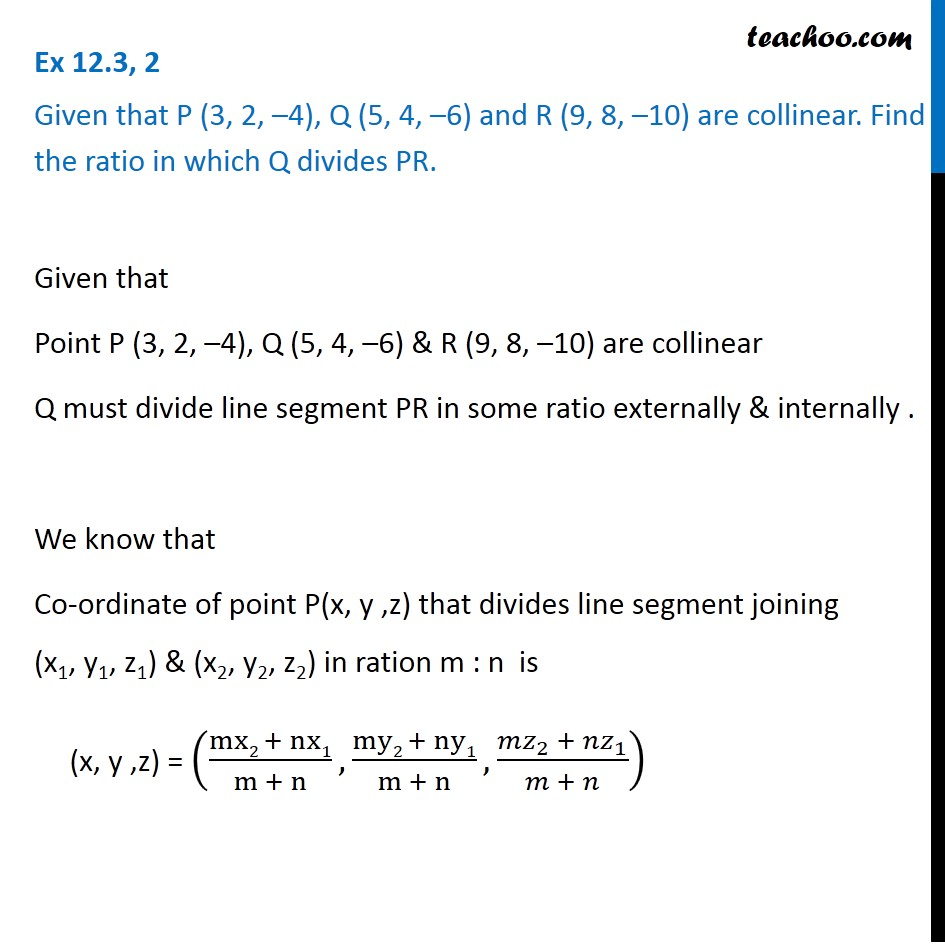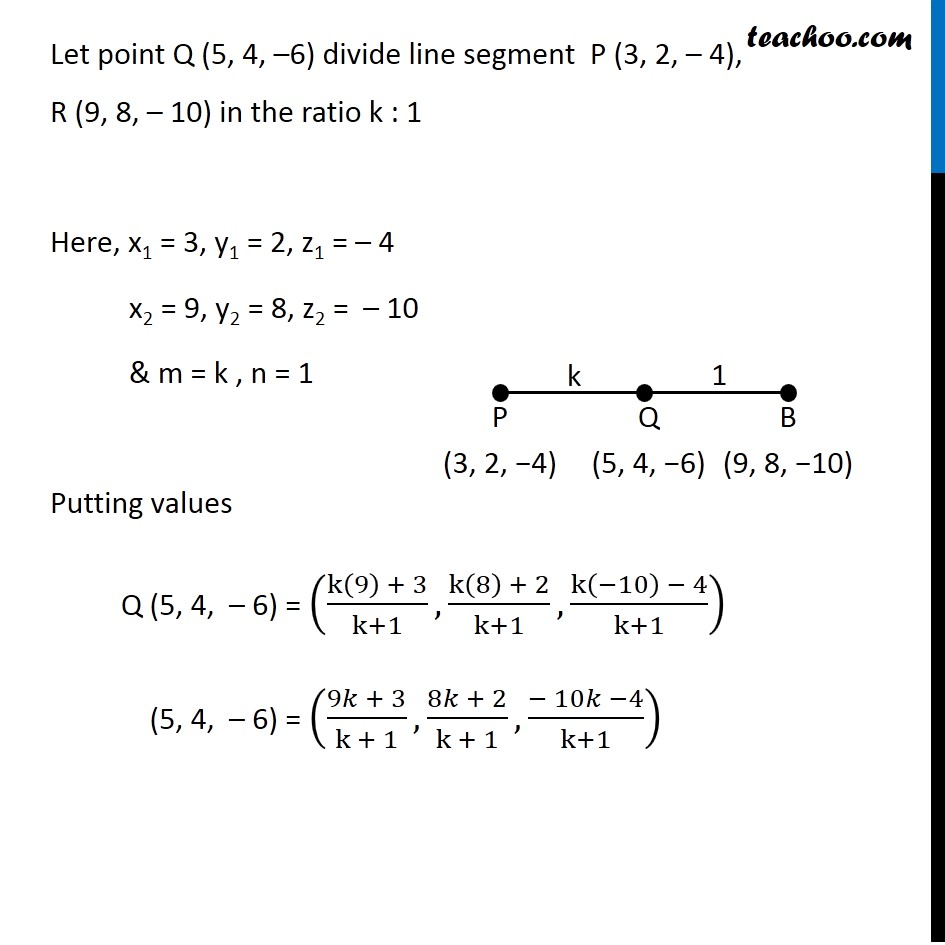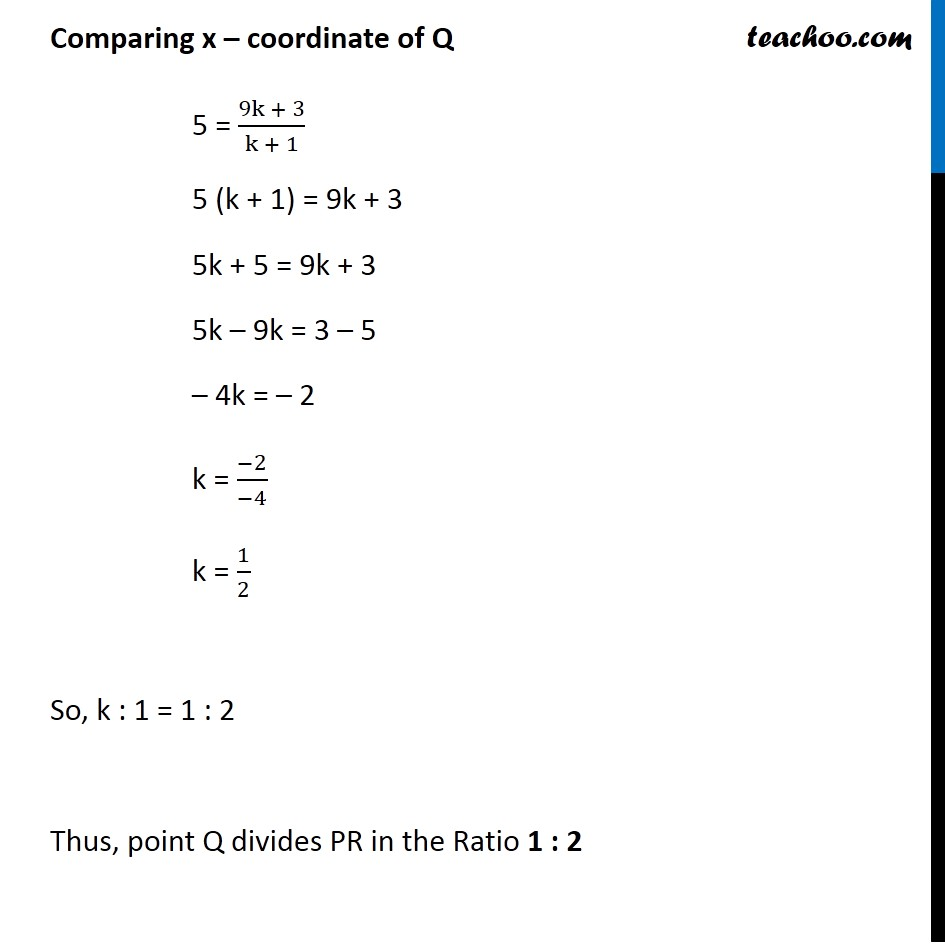Section Formula in 3D Geometry

Chapter 11 Class 11 - Intro to Three Dimensional Geometry
Serial order wiseLearn in your speed, with individual attention - Teachoo Maths 1-on-1 Class

### Transcript

Question 2 Given that P (3, 2, –4), Q (5, 4, –6) and R (9, 8, –10) are collinear. Find the ratio in which Q divides PR. Given that Point P (3, 2, –4), Q (5, 4, –6) & R (9, 8, –10) are collinear Q must divide line segment PR in some ratio externally & internally . We know that Co-ordinate of point P(x, y ,z) that divides line segment joining (x1, y1, z1) & (x2, y2, z2) in ration m : n is (x, y ,z) = ((mx2 + nx1)/(m + n),(my2 + ny1)/(m + n), (〖𝑚𝑧〗_2 + 〖𝑛𝑧〗_1)/(𝑚 + 𝑛)) Let point Q (5, 4, –6) divide line segment P (3, 2, – 4), R (9, 8, – 10) in the ratio k : 1 Here, x1 = 3, y1 = 2, z1 = – 4 x2 = 9, y2 = 8, z2 = – 10 & m = k , n = 1 Putting values Q (5, 4, – 6) = ((k(9) + 3)/(k+1),(k(8) + 2)/(k+1),(k(−10) − 4)/(k+1)) (5, 4, – 6) = ((9𝑘 + 3)/(k + 1),(8𝑘 + 2)/(k + 1),(− 10𝑘 −4)/(k+1)) Comparing x – coordinate of Q 5 = (9k + 3)/(k + 1) 5 (k + 1) = 9k + 3 5k + 5 = 9k + 3 5k – 9k = 3 – 5 – 4k = – 2 k = (−2)/(−4) k = 1/2 So, k : 1 = 1 : 2 Thus, point Q divides PR in the Ratio 1 : 2